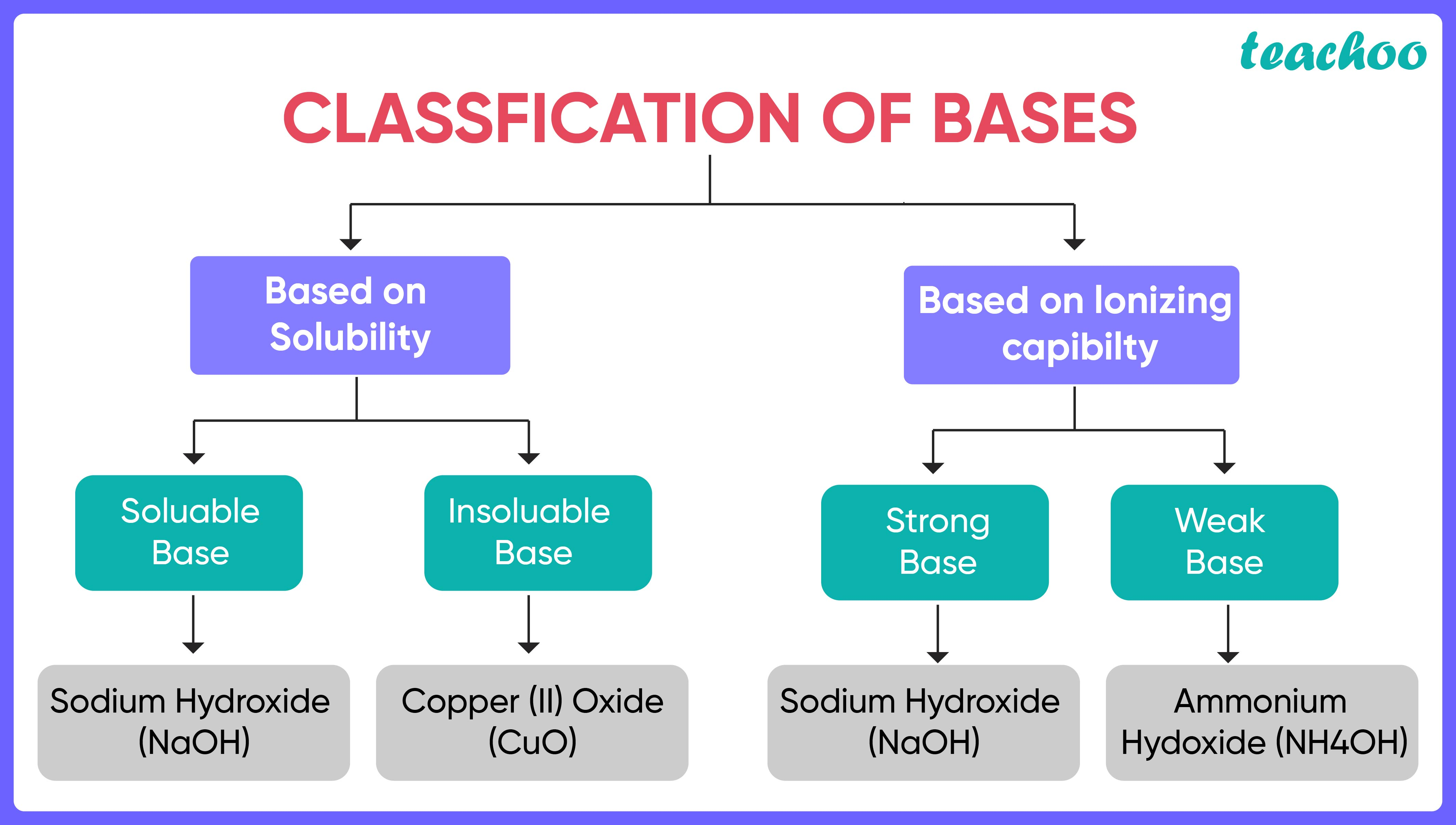Bases can be categorised based on different properties:## On the Basis of Solubility :

Bases can be classified as Soluble and Insoluble .

1. Soluble Bases : These are bases which are dissolvable in water. These are also called Alkalis . For Example : Sodium Hydroxide (NaOH), Potassium Hydroxide (KOH)

2. Insoluble Bases: These are bases which do not dissolve in water. For Example: Copper (II) Oxide (CuO).

## On the Basis of ionising capability:

Bases can be classified as Strong and Weak Bases.

1. Strong Bases : These are bases which completely dissociate (split into ions)  in water and thus produce a large number of Hydroxide (OH - ) ions. For Example : Potassium Hydroxide (KOH) and Sodium Hydroxide (NaOH).

2. Weak Bases : These are the bases which partially dissociate (split into ions) in water and produce relatively lesser numbers of Hydroxide (OH - ) ions. For Example : Ammonium Hydroxide (NH 4 OH) and Ammonia (NH 3 )

1. Class 10
2. Chapter 2 Class 10 - Acids, Bases and Salts (Term 1)
3. Concepts